# The right triangle altitude theorem - math problems

The altitude to the hypotenuse is the geometric mean of the two segments of the hypotenuse. Each leg of the right triangle is the mean proportional of the hypotenuse and the segment of the hypotenuse that is adjacent to the leg.

$h=\sqrt{{c}_{1}{c}_{2}}$

Also known as a geometric mean theorem. The geometric mean theorem is a special case of the chord theorem.

#### Number of problems found: 48

• Touch circle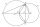Point A has a distance IA, kl = 10 cm from a circle k with radius r = 4 cm and center S. Calculate: a) the distance of point A from the point of contact T if the tangent to the circle is drawn from point A b) the distance of the contact point T from the l
• MIT 1869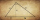You know the length of hypotenuse parts 9 and 16, at which the hypotenuse of a right triangle is divided by a perpendicular running from its opposite vertex. The task is to find the lengths of the sides of the triangle and the length of the line x. This a
• The bomber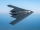From what distance in front of the target must a parachute load be dropped from an aircraft flying at an altitude of 1260 m if it slopes at a speed of 5.6 m/s and at the same time is carried in the direction of movement at a speed of 12 m/s. What is the d
• Altitude anglesCities A, B, C lie in one elevation plane. C is 50 km east of B, B is north of A. C is deviated by 50° from A. The plane flies around places A, B, C at the same altitude. When the aircraft is flying around B, its altitude angle to A is 12°. Find the altit
• Height of right RT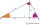The right triangle ABC has a hypotenuse c 9 cm long and a part of the hypotenuse cb = 3 cm. How long is the height of this right triangle?
• An observerAn observer standing west of the tower sees its top at an altitude angle of 45 degrees. After moving 50 meters to the south, he sees its top at an altitude angle of 30 degrees. How tall is the tower?
• Right triangle - ratioThe lengths of the legs of the right triangle ABC are in ratio b = 2: 3. The hypotenuse is 10 cm long. Calculate the lengths of the legs of that triangle.
• RT triangle and heightCalculate the remaining sides of the right triangle if we know side b = 4 cm long and height to side c h = 2.4 cm.
• Free space in the gardenThe grandfather's free space in the garden was in the shape of a rectangular triangle with 5 meters and 12 meters in length. He decided to divide it into two parts and the height of the hypotenuse. For the smaller part creates a rock garden, for the large
• Squares above sidesTwo squares are constructed on two sides of the ABC triangle. The square area above the BC side is 25 cm2. The height vc to the side AB is 3 cm long. The heel P of height vc divides the AB side in a 2: 1 ratio. The AC side is longer than the BC side. Calc
• Medians in right triangleIt is given a right triangle, angle C is 90 degrees. I know it medians t1 = 8 cm and median t2 = 12 cm. .. How to calculate the length of the sides?
• Isosceles triangle 9Given an isosceles triangle ABC where AB= AC. The perimeter is 64cm, and the altitude is 24cm. Find the area of the isosceles triangle.
• RT sidesFind the sides of a rectangular triangle if legs a + b = 17cm and the radius of the written circle ρ = 2cm.
• Spruce heightHow tall was spruce that was cut at an altitude of 8m above the ground and the top landed at a distance of 15m from the heel of the tree?
• Right isosceles triangleRight isosceles triangle has an altitude x drawn from the right angle to the hypotenuse dividing it into 2 equal segments. The length of one segment is 5 cm. What is the area of the triangle?
• Right 24Right isosceles triangle has an altitude x drawn from the right angle to the hypotenuse dividing it into 2 unequal segments. The length of one segment is 5 cm. What is the area of the triangle? Thank you.
• ParatrooperAfter the parachute is opened, the paratrooper drops to the ground at a constant speed of 2 m/s, with the sidewinding at a steady speed of 1.5 m/s. Find: a) the magnitude of its resulting velocity concerning the ground, b) the distance of his land from a
• Same area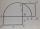There is a given triangle. Construct a square of the same area.
• Hypotenuse - RTA triangle has a hypotenuse of 55 and an altitude to the hypotenuse of 33. What is the area of the triangle?
• Conical areaA right angled triangle has sides a=12 and b=19 in right angle. The hypotenuse is c. If the triangle rotates on the c side as axis, find the volume and surface area of conical area created by this rotation.

Do you have an exciting math question or word problem that you can't solve? Ask a question or post a math problem, and we can try to solve it.

We will send a solution to your e-mail address. Solved examples are also published here. Please enter the e-mail correctly and check whether you don't have a full mailbox.